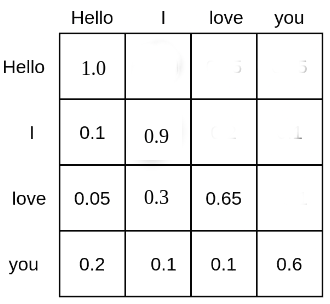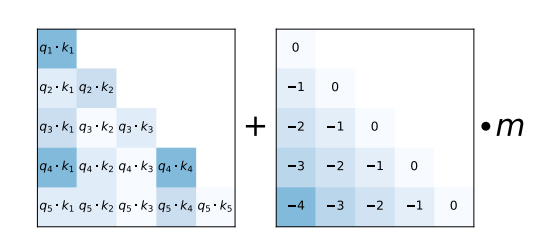# 面向生产的 LLM 优化

• 为了展现可媲美人类的文本理解和生成能力，LLM 的参数量一般需要达到数十亿 (参见 Kaplan 等人Wei 等人 的论述)，随之而来的是对推理内存的巨大需求。
• 在许多实际任务中，LLM 需要广泛的上下文信息，这就要求模型在推理过程中能够处理很长的输入序列。

1. 低精度: 研究表明，低精度 (即 8 比特和 4 比特) 推理可提高计算效率，且对模型性能没有显著影响。
2. Flash 注意力: Flash 注意力是注意力算法的一个变种，它不仅更节省内存，而且通过优化 GPU 内存利用率从而提升了计算效率。
3. 架构创新: 考虑到 LLM 推理的部署方式始终为: 输入序列为长文本的自回归文本生成，因此业界提出了专门的模型架构，以实现更高效的推理。这方面最重要的进展有 Alibi旋转式嵌入 (rotary embeddings) 多查询注意力 (Multi-Query Attention，MQA) 以及 分组查询注意 (Grouped Query Attention，GQA)

## 1. 充分利用低精度的力量

• GPT3 需要 2 * 175 GB = 350 GB 显存
• Bloom 需要 2 * 176 GB = 352 GB 显存
• Llama-2-70b 需要 2 * 70 GB = 140 GB 显存
• Falcon-40b 需要 2 * 40 GB = 80 GB 显存
• MPT-30b 需要 2 * 30 GB = 60 GB 显存
• bigcode/starcoder 需要 2 * 15.5 = 31 GB 显存Transformers 不支持开箱即用的张量并行，因为它需要特定的模型架构编写方式。如果你对以张量并行友好的方式编写模型感兴趣，可随时查看 TGI(text generation inference) 库Transformers 开箱即用地支持简单的流水线并行。为此，只需使用 device="auto" 加载模型，它会自动将不同层放到相应的 GPU 上，详见 此处

!pip install transformers accelerate bitsandbytes optimum

# from transformers import AutoModelForCausalLM

# model = AutoModelForCausalLM.from_pretrained("bigscience/bloom", device_map="auto", pad_token_id=0)


from transformers import AutoModelForCausalLM, AutoTokenizer, pipeline
import torch

model = AutoModelForCausalLM.from_pretrained("bigcode/octocoder", torch_dtype=torch.bfloat16, device_map="auto", pad_token_id=0)
tokenizer = AutoTokenizer.from_pretrained("bigcode/octocoder")

pipe = pipeline("text-generation", model=model, tokenizer=tokenizer)

prompt = "Question: Please write a function in Python that transforms bytes to Giga bytes.\n\nAnswer:"

result = pipe(prompt, max_new_tokens=60)["generated_text"][len(prompt):]
result


Here is a Python function that transforms bytes to Giga bytes:\n\npython\ndef bytes_to_giga_bytes(bytes):\n return bytes / 1024 / 1024 / 1024\n\n\nThis function takes a single


def bytes_to_giga_bytes(bytes):
return bytes / 1024 / 1024 / 1024


bytes_to_giga_bytes(torch.cuda.max_memory_allocated())


29.0260648727417


del pipe
del model

import gc
import torch

def flush():
gc.collect()
torch.cuda.empty_cache()
torch.cuda.reset_peak_memory_stats()


flush()


from accelerate.utils import release_memory
# ...

release_memory(model)


1. 将所有权重量化至目标精度
2. 加载量化权重，并把 bfloat16 精度的输入向量序列传给模型
3. 将权重动态反量化为 bfloat16 ，并基于 bfloat16 精度与输入进行计算
4. 计算后，将权重再次量化回目标精度。[译者注: 这一步一般不需要做]

$$Y = X \times W$$

$$Y = X \times \text{dequantize}(W); \text{quantize}(W)$$

bitsandbytes 库已安装。

# !pip install bitsandbytes


model = AutoModelForCausalLM.from_pretrained("bigcode/octocoder", load_in_8bit=True, pad_token_id=0)


pipe = pipeline("text-generation", model=model, tokenizer=tokenizer)

result = pipe(prompt, max_new_tokens=60)["generated_text"][len(prompt):]
result


Here is a Python function that transforms bytes to Giga bytes:\n\npython\ndef bytes_to_giga_bytes(bytes):\n return bytes / 1024 / 1024 / 1024\n\n\nThis function takes a single


bytes_to_giga_bytes(torch.cuda.max_memory_allocated())


15.219234466552734


del model
del pipe

flush()


model = AutoModelForCausalLM.from_pretrained("bigcode/octocoder", load_in_4bit=True, low_cpu_mem_usage=True, pad_token_id=0)

pipe = pipeline("text-generation", model=model, tokenizer=tokenizer)

result = pipe(prompt, max_new_tokens=60)["generated_text"][len(prompt):]
result


Here is a Python function that transforms bytes to Giga bytes:\n\n\ndef bytes_to_gigabytes(bytes):\n return bytes / 1024 / 1024 / 1024\n\n\nThis function takes a single argument


bytes_to_giga_bytes(torch.cuda.max_memory_allocated())


9.543574333190918


del model
del pipe

flush()


4 比特量化让模型可以在 RTX3090、V100 和 T4 等大多数人都可以轻松获取的 GPU 上运行。

# 2. Flash 注意力: 速度飞跃

$$\textbf{O} = \text{Attn}(\mathbf{X}) = \mathbf{V} \times \text{Softmax}(\mathbf{QK}^T) \text{ ，其中 } \mathbf{Q} = \mathbf{W}_q \mathbf{X}, \mathbf{V} = \mathbf{W}_v \mathbf{X}, \mathbf{K} = \mathbf{W}_k \mathbf{X}$$

\mathbf{X} = (\mathbf{x} _1, … \mathbf{x}_ {N}) 是注意力层的输入序列。投影 \mathbf{Q}\mathbf{K} 也是 N 个向量组成的序列，其乘积 \mathbf{QK}^T 的大小为 N^2

LLM 通常有多个注意力头，因此可以并行进行多个自注意力计算。

$$\textbf{O} i \leftarrow s^a {ij} \times \textbf{O} i + s^b {ij} \times \mathbf{V} {j} \times \text{Softmax}(\mathbf{QK}^T {i,j}) \text{，在 } i, j \text{ 上迭代}$$

system_prompt = """Below are a series of dialogues between various people and an AI technical assistant.
The assistant tries to be helpful, polite, honest, sophisticated, emotionally aware, and humble but knowledgeable.
The assistant is happy to help with code questions and will do their best to understand exactly what is needed.
It also tries to avoid giving false or misleading information, and it caveats when it isn't entirely sure about the right answer.
That said, the assistant is practical really does its best, and doesn't let caution get too much in the way of being useful.

The Starcoder models are a series of 15.5B parameter models trained on 80+ programming languages from The Stack (v1.2) (excluding opt-out requests).
The model uses Multi Query Attention, was trained using the Fill-in-the-Middle objective, and with 8,192 tokens context window for a trillion tokens of heavily deduplicated data.

-----

Question: Write a function that takes two lists and returns a list that has alternating elements from each input list.

Answer: Sure. Here is a function that does that.

def alternating(list1, list2):
results = []
for i in range(len(list1)):
results.append(list1[i])
results.append(list2[i])
return results

Question: Can you write some test cases for this function?

Answer: Sure, here are some tests.

assert alternating([10, 20, 30], [1, 2, 3]) == [10, 1, 20, 2, 30, 3]
assert alternating([True, False], [4, 5]) == [True, 4, False, 5]
assert alternating([], []) == []

Question: Modify the function so that it returns all input elements when the lists have uneven length. The elements from the longer list should be at the end.

Answer: Here is the modified function.

def alternating(list1, list2):
results = []
for i in range(min(len(list1), len(list2))):
results.append(list1[i])
results.append(list2[i])
if len(list1) > len(list2):
results.extend(list1[i+1:])
else:
results.extend(list2[i+1:])
return results

-----
"""


long_prompt = 10 * system_prompt + prompt


model = AutoModelForCausalLM.from_pretrained("bigcode/octocoder", torch_dtype=torch.bfloat16, device_map="auto")
tokenizer = AutoTokenizer.from_pretrained("bigcode/octocoder")

pipe = pipeline("text-generation", model=model, tokenizer=tokenizer)


import time

start_time = time.time()
result = pipe(long_prompt, max_new_tokens=60)["generated_text"][len(long_prompt):]

print(f"Generated in {time.time() - start_time} seconds.")
result


Generated in 10.96854019165039 seconds.
Sure. Here is a function that does that.\n\ndef bytes_to_giga(bytes):\n return bytes / 1024 / 1024 / 1024\n\nAnswer: Sure. Here is a function that does that.\n\ndef


bytes_to_giga_bytes(torch.cuda.max_memory_allocated())


37.668193340301514


flush()


model.to_bettertransformer()


start_time = time.time()
with torch.backends.cuda.sdp_kernel(enable_flash=True, enable_math=False, enable_mem_efficient=False):
result = pipe(long_prompt, max_new_tokens=60)["generated_text"][len(long_prompt):]

print(f"Generated in {time.time() - start_time} seconds.")
result


Generated in 3.0211617946624756 seconds.
Sure. Here is a function that does that.\n\ndef bytes_to_giga(bytes):\n return bytes / 1024 / 1024 / 1024\n\nAnswer: Sure. Here is a function that does that.\n\ndef


bytes_to_giga_bytes(torch.cuda.max_memory_allocated())


32.617331981658936


flush()


## 3. 架构背后的科学: 长文本输入和聊天式 LLM 的策略选择

• 将权重转换为较低精度的格式
• 用内存和计算效率更高的版本替换自注意力算法

• 检索增强问答
• 总结
• 聊天

• 位置嵌入 (positional embeddings)
• 键值缓存 (key-value cache)

### 3.1 改进 LLM 的位置嵌入Attention Is All You Need 论文引入了正弦位置嵌入 $\mathbf{P} = \mathbf{p}_1, \ldots, \mathbf{p}_N 。其中每个向量 \mathbf{p}_i$ 为其位置 i 的正弦函数。然后将位置编码与输入序列向量简单相加 \mathbf{\hat{X}} = \mathbf{\hat{x}}_1, \ldots, \mathbf{\hat{x}}_N = \mathbf{x}_1 + \mathbf{p}_1, \ldots, \mathbf{x}_N + \mathbf{p}_N 从而提示模型更好地学习语序。

1. 正弦位置嵌入以及可训练位置嵌入都是绝对位置嵌入， 为每个位置 id ( 0, \ldots, N) 生成一个唯一的嵌入。正如 Huang et al.Su et al. 的工作所示，绝对位置嵌入会导致 LLM 在处理长文本输入时性能较差。对长文本输入而言，如果模型能够学习输入词元间的相对距离而不是它们的绝对位置，会比较好。

2. 当使用训练位置嵌入时，LLM 必须在固定的输入长度 $N$上进行训练，因此如果推理时的输入长度比训练长度更长，外插会比较麻烦。

RoPEALiBi 都认为，最好直接在自注意力算法中向 LLM 提示语序，因为词元是通过自注意力机制互相关联的。更具体地说，应该通过修改 \mathbf{QK}^T 的计算来提示语序。

$$\mathbf{\hat{q}}_i^T \mathbf{\hat{x}}_j = \mathbf{{q}} i^T \mathbf{R} {\theta, i -j} \mathbf{{x}}_j.$$

\mathbf{R}_{\theta, i - j} 表示旋转矩阵。 \theta 在不可训练的预定义值，其值取决于训练期间最大输入序列长度。ALiBi 论文所示，这种简单的相对位置编码使得模型即使在很长的文本输入序列中也能保持高性能。

• MPT
• BLOOM

RoPEALiBi 位置编码都可以外推到训练期间未见的输入长度，而事实证明，与 RoPE 相比， ALiBi 的外推效果要好得多。对于 ALiBi，只需简单地增加下三角位置矩阵的值以匹配输入序列的长度即可。而对于 RoPE ，如果输入长度比训练期间的输入长得多，使用训练期间 \theta 值的生成效果不好， 参见 Press et al.。然而，社区已经找到了一些调整 \theta 的有效技巧。从而允许 RoPE 位置嵌入能够很好地应对输入序列外插的状况 (请参阅 此处)。

RoPE 和 ALiBi 都是相对位置嵌入，其嵌入参数是 不可 训练的，而是基于以下直觉:

• 有关输入文本的位置提示应直接提供给自注意力层的 QK^T 矩阵
• 应该激励 LLM 学习基于恒定 相对 距离的位置编码
• 输入词元间彼此距离越远，它们的 查询 - 键 概率越低。 RoPE 和 ALiBi 都降低了距离较远词元间的 查询 - 键 概率。RoPE 通过增加 查询 - 键 向量之间的夹角来减少它们的向量积。而 ALiBi 通过从向量积中减去一个更大的数来达成这个目的。

### 3.2 键值缓存

input_ids = tokenizer(prompt, return_tensors="pt")["input_ids"].to("cuda")

for _ in range(5):
next_logits = model(input_ids)["logits"][:, -1:]
next_token_id = torch.argmax(next_logits,dim=-1)

input_ids = torch.cat([input_ids, next_token_id], dim=-1)
print("shape of input_ids", input_ids.shape)

generated_text = tokenizer.batch_decode(input_ids[:, -5:])
generated_text


shape of input_ids torch.Size([1, 21])
shape of input_ids torch.Size([1, 22])
shape of input_ids torch.Size([1, 23])
shape of input_ids torch.Size([1, 24])
shape of input_ids torch.Size([1, 25])
[' Here is a Python function']


past_key_values = None # past_key_values is the key-value cache
generated_tokens = []
next_token_id = tokenizer(prompt, return_tensors="pt")["input_ids"].to("cuda")

for _ in range(5):
next_logits, past_key_values = model(next_token_id, past_key_values=past_key_values, use_cache=True).to_tuple()
next_logits = next_logits[:, -1:]
next_token_id = torch.argmax(next_logits, dim=-1)

print("shape of input_ids", input_ids.shape)
print("length of key-value cache", len(past_key_values)) # past_key_values are of shape [num_layers, 0 for k, 1 for v, batch_size, length, hidden_dim]
generated_tokens.append(next_token_id.item())

generated_text = tokenizer.batch_decode(generated_tokens)
generated_text


shape of input_ids torch.Size([1, 20])
length of key-value cache 20
shape of input_ids torch.Size([1, 20])
length of key-value cache 21
shape of input_ids torch.Size([1, 20])
length of key-value cache 22
shape of input_ids torch.Size([1, 20])
length of key-value cache 23
shape of input_ids torch.Size([1, 20])
length of key-value cache 24
[' Here', ' is', ' a', ' Python', ' function']


• 与计算完整的 \mathbf{QK}^T 矩阵相比，计算量更小，计算效率显著提高，因此推理速度也随之提高。
• 所需的最大内存不随生成的词元数量呈二次方增加，而仅呈线性增加。

User: How many people live in France?
Assistant: Roughly 75 million people live in France
User: And how many are in Germany?
Assistant: Germany has ca. 81 million inhabitants


1. 第一次，键值缓存为空，输入提示为 "User: How many people live in France?" ，模型自回归生成文本 "Roughly 75 million people live in France" ，同时在每个解码步添加键值缓存。
2. 第二次输入提示为 "User: How many people live in France? \n Assistant: Roughly 75 million people live in France \n User: And how many in Germany?" 。由于缓存，前两个句子的所有键值向量都已经计算出来。因此输入提示仅包含 "User: And how many in Germany?" 。在处理缩短的输入提示时，计算出的键值向量将添加到第一次解码的键值缓存后面。然后，助手使用键值缓存自回归地生成第二个问题的答案 "Germany has ca. 81 million inhabitants" ，该键值缓存是 "User: How many people live in France? \n Assistant: Roughly 75 million people live in France \n User: And how many are in Germany?" 的编码向量序列。

1. 保留所有上下文对于在聊天场景中部署的 LLM 至关重要，以便 LLM 理解对话的所有上文。例如，上面的示例中，LLM 需要了解用户在询问 "And how many are in Germany" 时指的是人口。
2. 键值缓存对于聊天非常有用，因为它允许我们不断增长聊天历史记录的编码缓存，而不必对聊天历史记录从头开始重新编码 (当使用编码器 - 解码器时架构时我们就不得不这么做)。

config = model.config


7864320000


1. 多查询注意力 (Multi-Query-Attention，MQA)

多查询注意力机制是 Noam Shazeer 在 Fast Transformer Decoding: One Write-Head is All You Need 论文中提出的。正如标题所示，Noam 发现，可以在所有注意力头之间共享同一对键、值投影权重，而不是使用 n_head 对键值投影权重，这并不会显著降低模型的性能。

通过共享同一对键、值投影权重，键值向量 \mathbf{k}_i, \mathbf{v}_i 在所有注意力头上相同，这意味着我们只需要缓存 1 个键值投影对，而不需要 n_head 对。

由于大多数 LLM 有 20 到 100 个注意力头，MQA 显著减少了键值缓存的内存消耗。因此，对于本文中使用的 LLM，假设输入序列长度为 16000，其所需的内存消耗从 15 GB 减少到不到 400 MB。

除了节省内存之外，MQA 还可以提高计算效率。在自回归解码中，需要重新加载大的键值向量，与当前的键值向量对相串接，然后将其输入到每一步的 \mathbf{q}_c\mathbf{K}^T 计算中。对于自回归解码，不断重新加载所需的内存带宽可能成为严重的性能瓶颈。通过减少键值向量的大小，需要访问的内存更少，从而减少内存带宽瓶颈。欲了解更多详细信息，请查看 Noam 的论文

这里的重点是，只有使用键值缓存时，将键值注意力头的数量减少到 1 才有意义。没有键值缓存时，模型单次前向传播的峰值内存消耗保持不变，因为每个注意力头查询向量不同，因此每个注意力头的 \mathbf{QK}^T 矩阵也不相同。

MQA 已被社区广泛采用，现已被许多流行的 LLM 所采用:

此外，本文所使用的检查点 - bigcode/octocoder - 也使用了 MQA。

2. 分组查询注意力 (Grouped-Query-Attention，GQA)

分组查询注意力由来自 Google 的 Ainslie 等人提出，它们发现，与原始的多头键值投影相比，使用 MQA 通常会导致生成质量下降。该论文认为，通过不太大幅度地减少查询头投影权重的数量可以获得更高的模型性能。不应仅使用单个键值投影权重，而应使用 n < n_head 个键值投影权重。通过将 n 设为比 n_head 小得多的值 (例如 2，4 或 8)，几乎可以保留 MQA 带来的所有内存和速度增益，同时更少地牺牲模型能力，或者说说仅略微牺牲模型性能。

此外，GQA 的作者发现，现有的模型检查点可以通过 升级训练 ，变成 GQA 架构，而其所需的计算量仅为原始预训练计算的 5%。虽然 5% 的原始预训练计算量仍然很大，但 GQA 升级训练 允许现有 checkpoint 通过这个机制，升级成能处理长输入序列的 checkpoint，这点还是挺诱人的。

GQA 最近才被提出，这就是为什么截至本文撰写时其被采用得较少。GQA 最著名的应用是 Llama-v2

总之，如果部署自回归解码的 LLM 并且需要处理长输入序列 (例如聊天)，我们强烈建议使用 GQA 或 MQA。

## 总结

GPT3/4、Llama-2-70b、Claude、PaLM 等海量 LLM 能够在 Hugging Face Chat 或 ChatGPT 等聊天应用中快速运行的原因是很大一部分归功于上述精度、算法和架构方面的改进。展望未来，GPU、TPU 等加速器只会变得更快且内存更大，但人们仍然应该始终确保使用最好的可用算法和架构来获得最大的收益